Smartick is a fun way to learn math!May08

# Logic Exercises at Smartick and a Math Riddle

Hello! Today at Smartick we are going to review one of the most popular topics among students: logic.

First, try to solve the famous riddle that went viral a few months ago!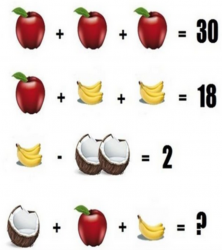That is the same curiosity our students feel when they complete their sessions.

Logic, as such, is a branch of mathematics that is not usually worked on extensively in school. Logical reasoning is not only useful to us in everyday situations, but it is the basis for the orderly reasoning that allows us to reach elaborate conclusions.

In fact, it is not just a branch of mathematics, but what mathematics is based on.  When we work on any mathematical content we are working on logical reasoning.  However, working separately on logic helps serves as different and compelling content. So in Smartick, we have logic sessions that are separate from our “normal” sessions. The students work with them depending on how they do and without a time limit on the exercises.

So … what are these exercises that our children like so much?  That is the purpose of today’s post, to teach different areas that work within the logic sessions.

Try to solve some of them!

### Attention Exercises

These exercises work with the attention span of our students. Thus, they should be very careful to choose the way in which two images are equal: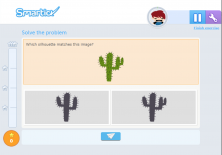or to follow the correct path: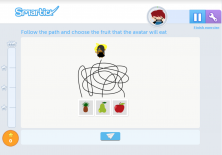### Spatial Exercises

These exercises are specially developed for children to exercise their spatial vision. They will have to undo, or breakdown, a figure to find what we call its development: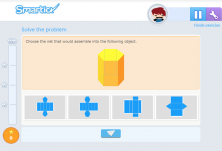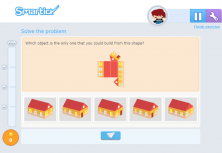You must mentally rotate the images to see which one is the same as the one given or mentally adjust its position: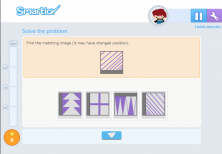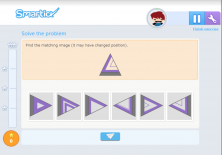### Numerical Exercises

These are exercises in which students will face some sort of reasoning that includes numbers or arithmetic operations. For example:

###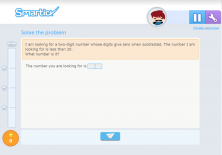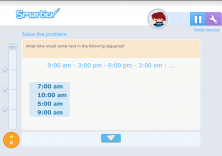### Verbal Exercises

In these exercises, students will use verbal logic to find synonyms, antonyms or words that do not fit.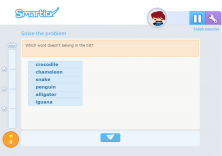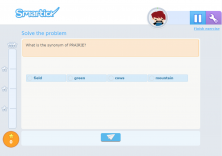### Propositions, riddles, deductions, etc.

They are the closest thing to what we find when we open a logic puzzle book. They have to make all kinds of deductions.  Of course, we will guide them from the easiest to the most difficult. In recent levels, we have logical questions not only for children but also for parents!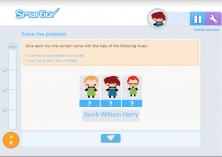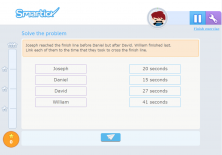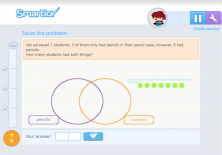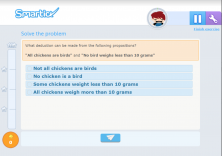And you’re done, we hope you liked it!  To enjoy even more you can begin a trial with Smartick and fun is guaranteed!

PS: I could not leave you without the solution to the fruit puzzle.

To begin,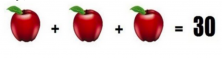So, each apple is worth 10, because 10 + 10 + 10 = 30.
Now we have,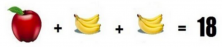If we remove the apple, we will have to move 10 to the other side so that the equation is balanced. With what we know, two bananas are equal to 8, so one banana equals 4. We use the following equation, 4 minus coconuts equals 2, so then coconuts are equal to 2.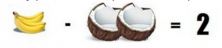In summary, we have:

• Apple = 10
• 4 bananas = 4
• 2 coconuts = 2

Notice the last equation,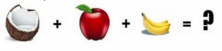We need to know how much 1 coconut and 3 bananas weigh. It’s easy because if 4 bananas are equal to 4,  that means that 1 banana equals 1, so 3 bananas are equal to 3.  Using that same reasoning if 2 coconuts weigh 2, then one coconut weighs 1.  Therefore, the information we have to put in the equation is:

• Coconut = 1
• Apple = 10
• 3 bananas = 3

That is, 1 + 10 + 3 = 14. This is the solution!